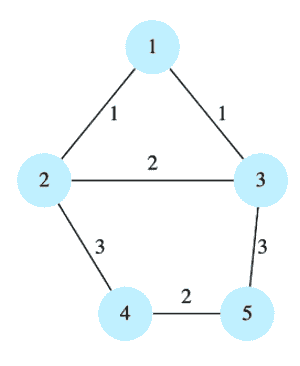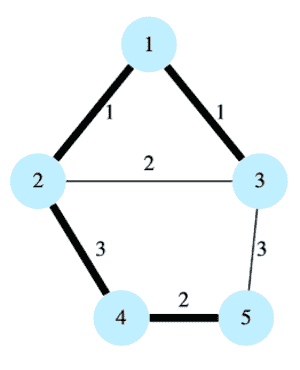# Minimum Spanning Tree

## Problem Statement

Find the minimum spanning tree of a connected, undirected graph with weighted edges.

Consider the following graph.The minimum spanning tree of the above graph would be:## Hint

• Minimum weight edge

## Try it yourself

#include <iostream>#include <vector>using namespace std;class vertex {private:  int id;  bool visited;public:  vertex(int id, bool visited) {    this->id = id;    this->visited = visited;  }  int getId() {    return id;  }  void setId(int id) {    this->id = id;  }  bool isVisited() {    return visited;  }  void setVisited(bool visited) {    this->visited = visited;  }};class edge {private:  int weight;  bool visited;  vertex* src;  vertex* dest;public:  edge(int weight, bool visited, vertex* src, vertex* dest){    this->weight = weight;    this->visited = visited;    this->src = src;    this->dest = dest;  }  int getWeight() const {    return weight;  }  void setWeight(int weight) {    this->weight = weight;  }    bool isVisited() const {    return visited;  }  void setVisited(bool visited) {    this->visited = visited;  }  vertex* getSrc() const {    return src;  }  void setSrc(vertex* src) {    this->src = src;  }  vertex* getDest() const {    return dest;  }  void setDest(vertex* dest) {    this->dest = dest;  }};class graph {private:  vector<vertex*> g;    //vertices  vector<edge*> e;      //edgespublic:  int find_min_spanning_tree(){        //TODO: Write - Your - Code  }    const vector<vertex*>& getG() const {    return g;  }  void setG(const vector<vertex*>& g) {    this->g = g;  }  const vector<edge*>& getE() const {    return e;  }  void setE(const vector<edge*>& e) {    this->e = e;  }  // This method returns the vertex with a given id if it  // already exists in the graph, returns NULL otherwise  vertex* vertex_exists(int id) {    for (int i = 0; i < this->g.size(); i++) {      if (g[i]->getId() == id) {        return g[i];      }    }    return nullptr;  }  string print_graph() {    string result = "";    for (int i = 0; i < g.size(); i++) {      cout<<g[i]->getId()<< ' ' <<g[i]->isVisited()<< endl;    }    cout << endl;    for (int i = 0; i < e.size(); i++) {      result += "[" + std::to_string(e[i]->getSrc()->getId()) + "->" + std::to_string(e[i]->getDest()->getId()) + "],";      cout << e[i]->getSrc()->getId() << "->"          << e[i]->getDest()->getId() << "["          << e[i]->getWeight() << ", "          << e[i]->isVisited() << "]  ";    }    cout << endl << endl;    return result;  }    // This method generates the graph with v vertices  // and e edges  void generate_graph(int vertices,      vector< vector<int> > edges) {    // create vertices    for (int i = 0; i < vertices; i++) {      vertex* v = new vertex(i + 1, false);      this->g.push_back(v);    }    // create edges    for (int i = 0; i < edges.size(); i++) {      vertex* src = vertex_exists(edges[i]);      vertex* dest = vertex_exists(edges[i]);      edge* e = new edge(edges[i], false, src, dest);      this->e.push_back(e);    }  }};

## Solution

#include <iostream>#include <vector>using namespace std;class vertex {  private:  int id;  bool visited;  public:  vertex(int id, bool visited) {    this->id = id;    this->visited = visited;  }  int getId() {    return id;  }  void setId(int id) {    this->id = id;  }  bool isVisited() {    return visited;  }  void setVisited(bool visited) {    this->visited = visited;  }};class edge {  private:  int weight;  bool visited;  vertex* src;  vertex* dest;  public:  edge(int weight, bool visited, vertex* src, vertex* dest){    this->weight = weight;    this->visited = visited;    this->src = src;    this->dest = dest;  }  int getWeight() const {    return weight;  }  void setWeight(int weight) {    this->weight = weight;  }  bool isVisited() const {    return visited;  }  void setVisited(bool visited) {    this->visited = visited;  }  vertex* getSrc() const {    return src;  }  void setSrc(vertex* src) {    this->src = src;  }  vertex* getDest() const {    return dest;  }  void setDest(vertex* dest) {    this->dest = dest;  }};class graph {  private:  vector<vertex*> g;    //vertices  vector<edge*> e;      //edges  public:  const vector<vertex*>& getG() const {    return g;  }  void setG(const vector<vertex*>& g) {    this->g = g;  }  const vector<edge*>& getE() const {    return e;  }  void setE(const vector<edge*>& e) {    this->e = e;  }  // This method returns the vertex with a given id if it  // already exists in the graph, returns NULL otherwise  vertex* vertex_exists(int id) {    for (int i = 0; i < this->g.size(); i++) {      if (g[i]->getId() == id) {        return g[i];      }    }    return nullptr;  }  // This method generates the graph with v vertices  // and e edges  void generate_graph(int vertices,                      vector< vector<int> > edges) {    // create vertices    for (int i = 0; i < vertices; i++) {      vertex* v = new vertex(i + 1, false);      this->g.push_back(v);    }    // create edges    for (int i = 0; i < edges.size(); i++) {      vertex* src = vertex_exists(edges[i]);      vertex* dest = vertex_exists(edges[i]);      edge* e = new edge(edges[i], false, src, dest);      this->e.push_back(e);    }  }  // This method finds the MST of a graph using  // Prim's Algorithm  // returns the weight of the MST  int find_min_spanning_tree(){    int vertex_count = 0;    int weight = 0;    // Add first vertex to the MST    vertex* current = g;    current->setVisited(true);    vertex_count++;    // Construct the remaining MST using the    // smallest weight edge    while(vertex_count < g.size()){      edge* smallest = NULL;      for(int i=0; i<e.size(); i++){        if(e[i]->isVisited() == false){          if (e[i]->getSrc()->isVisited() == true              && e[i]->getDest()->isVisited() == false) {                if (smallest == NULL) {                  smallest = e[i];                }                else if (e[i]->getWeight() < smallest->getWeight()) {                  smallest = e[i];                }          }        }      }      smallest->setVisited(true);      smallest->getDest()->setVisited(true);      weight += smallest->getWeight();      vertex_count++;    }    return weight;  }  void print_graph() {    for (int i = 0; i < g.size(); i++) {      cout << g[i]->getId() << ' ' << g[i]->isVisited()        << endl;    }    cout << endl;    for (int i = 0; i < e.size(); i++) {      cout << e[i]->getSrc()->getId() << "->"        << e[i]->getDest()->getId() << "["        << e[i]->getWeight() << ", "        << e[i]->isVisited() << "]  ";    }    cout << endl << endl;  }  void print_mst(int w){    cout << "MST\n";    for(int i=0; i<e.size(); i++){      if(e[i]->isVisited() == true){        cout << e[i]->getSrc()->getId() << "->"          << e[i]->getDest()->getId() << endl;      }    }    cout << "weight: " << w << endl;    cout << endl;  }};void test_graph1() {  graph g;  int v = 5;  // each edge contains the following: weight, src, dest  vector<vector<int> > e = { { 1, 1, 2 }, { 1, 1, 3 }, { 2, 2,                                                        3 }, { 3, 2, 4 }, { 3, 3, 5 }, { 2, 4, 5 } };  g.generate_graph(v, e);  g.print_graph();  cout << "Testing graph 1...\n";  //g.print_graph();  int w = g.find_min_spanning_tree();  g.print_mst(w);}void test_graph2() {  graph g;  int v = 7;  // each edge contains the following: weight, src, dest  vector<vector<int> > e = { { 2, 1, 4 }, { 1, 1, 3 }, { 2, 1,                                                        2 }, { 1, 3, 4 }, { 3, 2, 4 }, { 2, 3, 5 }, { 2, 4, 7 },                            { 1, 5, 6 }, { 2, 5, 7 } };  g.generate_graph(v, e);  cout << "Testing graph 2...\n";  //g.print_graph();  int w = g.find_min_spanning_tree();  g.print_mst(w);}int main() {  test_graph1();  test_graph2();  cout << "Completed!" << endl;  return 0;}

## Solution Explanation

### Runtime Complexity

Quadratic, $O(n^{2})$

Here, ‘n’ is the number of vertices.

### Memory Complexity

Linear, O(n + e)

Here, ‘n’ is the number of vertices and ‘e’ is the number of edges.

### Solution Breakdown

A spanning tree of a connected, undirected graph is a subgraph that is a tree and connects all the vertices together. One graph can have many different spanning trees. A graph with n vertices has a spanning tree with n-1 edges.

A weight can be assigned to each edge of the graph. The weight of a spanning tree is the sum of weights of all the edges of the spanning tree. A minimum spanning tree (MST) for a weighted, connected and undirected graph is a spanning tree with a weight less than or equal to the weight of every other spanning tree.

We’ll find the minimum spanning tree of a graph using Prim’s algorithm. This algorithm builds the tree one vertex at a time, starting from any arbitrary vertex. It adds the minimum weight edge from the tree being constructed to a vertex from the remaining vertices at each step.

The algorithm is as follows:

Initialize the MST with an arbitrary vertex from the graph
Find the minimum weight edge from the constructed graph to the vertices not yet added in the graph
Add that edge and vertex to the MST
Repeat steps 2-3 until all the vertices have been added to the MST


The time complexity to find the minimum weight edge is $O(n^{2})$. However, it can be improved further by using heaps to find the minimum weight edge.

Practice problems like this and many more by checking out our Grokking the Coding Interview: Patterns for Coding Questions course!

Learn in-demand tech skills in half the time